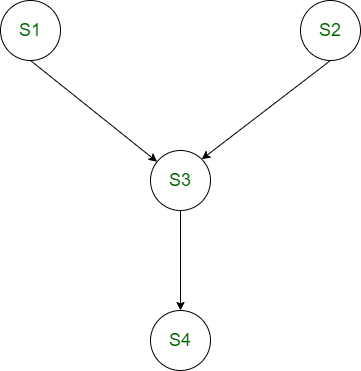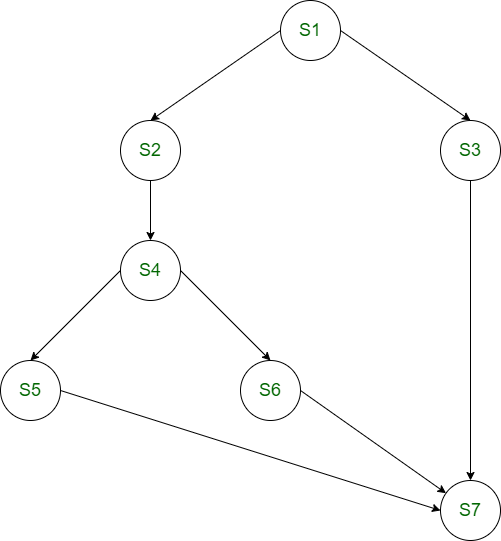# Precedence Graph in Operating System

• Difficulty Level : Basic
• Last Updated : 05 Jun, 2020

Prerequisite – Process Synchronization
Precedence Graph is a directed acyclic graph which is used to show the execution level of several processes in operating system. It consists of nodes and edges. Nodes represent the processes and the edges represent the flow of execution.

Properties of Precedence Graph :
Following are the properties of Precedence Graph:

• It is a directed graph.
• It is an acyclic graph.
• Nodes of graph correspond to individual statements of program code.
• Edge between two nodes represents the execution order.
• A directed edge from node A to node B shows that statement A executes first and then Statement B executes.

Consider he following code:

```S1 : a = x + y;
S2 : b = z + 1;
S3 : c = a - b;
S4 : w = c + 1;
```

If above code is executed concurrently, the following precedence relations exist:

• c = a – b cannot be executed before both a and b have been assigned values.
• w = c + 1 cannot be executed before the new values of c has been computed.
• The statements a = x + y and b = z + 1 could be executed concurrently.Example:
Consider the following precedence relations of a program:

1. S2 and S3 can be executed after S1 completes.
2. S4 can be executed after S2 completes.
3. S5 and S6 can be executed after S4 completes.
4. S7 can be executed after S5, S6 and S3 complete.

Solution:My Personal Notes arrow_drop_up